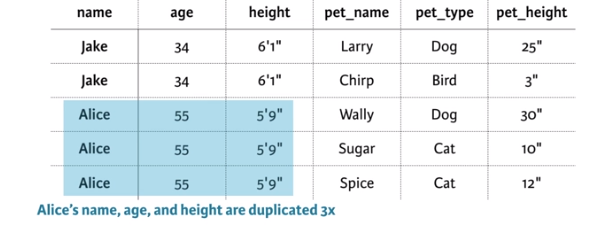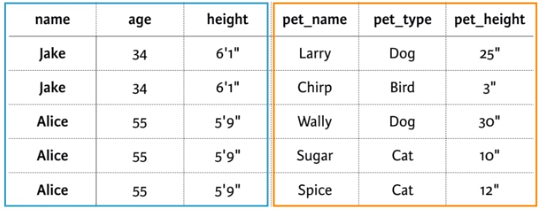Collect –> Clean –> Analyse –< Report

• Exploring raw data
• Tidying data
• Preparing data for analysis

## {tidyr} functions

It should be used when you have columns that are not variables and you want to collapse them into key-value pairs.

``gather(wide_df, my_key, my_val, -col)``
The opposite of gather() is spread(), which takes key-values pairs and spreads them across multiple columns.
This is useful when values in a column should actually be column names (i.e. variables). It can also make data more compact and easier to read.
`spread(long_df, my_key, my_val)`
Multiple values are stored in one column
``separate(df, col = Year_Mo, into = c("Year", "Month"), sep = "/")``
The opposite of separate() is unite(), which takes multiple columns and pastes them together.
sep=’_’ by default.
```unite(treatments, year_mo, year, month)
```

## When we call a data messy?

— Column headers are values (not var names)
This is often the case when working with repeated measures data, where measurements are taken on subjects of interest on multiple occasions over time.

` gf2 <- gather(gf, month, amount, -YEAR)`

# Arrange rows by YEAR using dplyr’s arrange

```df2 <- arrange(df2, YEAR)
- Multiple variables are stored in 1 column - use separate{tidyr}
- A single observation unit is stored in multiple tables
- Multiple types of observational units are stored in the same table - use spread(...)
- Multiple values are stored in one column - use separate(...)

# Separate the yr_month column into two
separate(df_long, yr_month, c("year","month"), "_" )
```

## One table: "People & Pets"## Two table### Column names are Values

Тhe column names X1-X31 represent days of the month,
which should really be values of a new variable called day.

```library(tidyr)
# Gather the columns
>gather(weather,day, value,X1:X31 , na.rm = TRUE)

X year month           measure day value
1 1 2014    12  Max.TemperatureF  X1    64
2 2 2014    12 Mean.TemperatureF  X1    52
3 3 2014    12  Min.TemperatureF  X1    39
4 4 2014    12    Max.Dew.PointF  X1    46
5 5 2014    12    MeanDew.PointF  X1    40
6 6 2014    12     Min.DewpointF  X1    26
```

### Values are Variables names

The unique values in 'Measure' column should each have its own column:

```library(tidyr)
# First remove column of row names
without_x <- weather2[, -1]

year month day CloudCover    Events Max.Dew.PointF Max.Gust.SpeedMPH
1 2014    12  X1          6      Rain             46                29
2 2014    12  X2          7 Rain-Snow             40                29
3 2014    12  X3          8      Rain             49                38
4 2014    12  X4          3                       24                33
5 2014    12  X5          5      Rain             37                26
6 2014    12  X6          8      Rain             45                25
Max.Humidity Max.Sea.Level.PressureIn Max.TemperatureF Max.VisibilityMiles
1           74                    30.45               64                  10
2           92                    30.71               42                  10
3          100                     30.4               51                  10
4           69                    30.56               43                  10
............
```

## Preparing Data for Analysis

Type of variables in R: Character, Numeric, Integer (1L), Factor (for categprical vars), Logical.

Common type conversions:

```as.character(2018)
as.numeric(TRUE)
as.integer(2018)
as.factor("string")
as.logical(1)
class("2018")
class(2018L)
class(factor(string))```

Understanding of the structure of data:
``````class()          #class of data object
dim()            #dimentions of data
names()          #column names
str()            #preview of data with helpful details
glimpse{dplyr}   #convenient version of str()
summary()        #quick look on distribution of the break down on each column on a data
``````

{lubridate} Coersion to Date:

``````library(lubridate)
# Parse as date
dmy("5 Nov 2018")
# Parse as date and time (with no seconds)
mdy_hm("November 5, 2018 18:34")

# Coerce to a date (with no time)
df\$date <- ymd(df\$date)

# Coerce
df\$date_and_time<- ymd_hm(df\$date)
``````
``````library(stringr)
# Trim all leading and trailing whitespace
str_trim(c("  this is  ", "a  ", "   test"))
``````toupper()
tolower()``````
{stringr} Finding and replacing strings
``````str_detect(c("banana", "kiwi"), "a")
  TRUE FALSE
str_replace(c("banana", "kiwi"), "a", "o")
 "bonana" "kiwi"``````
Finding and straightforward dealing with missing data & special values
Missing Data representation:
R Excel SPSS/SAS Other
NA
#N/A
single dot
empty string, -1, other special codes
Special values representation: Inf – Infinite value (may be as outliers indicators) NaN – not a number (1/0, 0/0) Finding Missing Data:
``````#Check for NAs
is.na(df)
#Are there any NAs?
any(is.na(df))
#Count NAs number
sum(is.na(df))
#Use summary(), table() to turn up unexpected values in data
summary(df)
#Identify the indices where the column1 has NAs
which(is.na(df\$column1))
#Find rows with no missing data
complete.cases(df)  #returns a logical value for each row
#Rows with no missing data:
df[complete.cases(df),]
#Removing any ROW with missing values
na.omit(df) ``````
Outliers – extreme values distant from other values. They may be – valid measurements, variability in measyrement, experimental error, data entry error..
Use summary(), hist() and boxplot() to get a first impression of outlier data.
Boxplot() draws a box around the middle 50% of values for a given variable, with a bolded horizontal line drawn at the median. Values that fall far from the bulk of the data points (i.e. outliers) are denoted by open circles.
``````library(tidyr)
library(dplyr)
library(stringr)
library(lubridate)

# Remove X's from day column
weather3\$day <- str_replace(weather3\$day, "X", "")

# Unite the year, month, and day columns
weather4 <- unite(weather3, date, year, month, day, sep = "-")
names(weather3)

# Convert date column to proper date format using lubridates's ymd()
weather4\$date <- ymd(weather4\$date)

# Rearrange columns using dplyr's select()
weather5 <- select(weather4, date, Events, col1:col5)
#Conveniently apply as.numeric() to all columns
weather6 <- mutate_at(weather5, vars(col1:col5), funs(as.numeric))
``````